# Small denominators

(diff) ← Older revision | Latest revision (diff) | Newer revision → (diff)

small divisors

Divisors of the form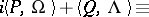(1)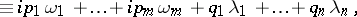which appear in the coefficients of series obtained when integrating differential equations using Taylor series, Fourier series or Poisson series; here,are integer vectors,is a real vector,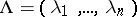is a complex vector, and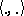denotes the scalar product. The existence of a solution and its properties, such as analyticity, smoothness, etc., depend essentially on the arithmetic nature of the numbers,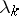and the same properties (analyticity, smoothness, etc.) of the differential equations. Conditions are given below which guarantee analyticity of solutions corresponding to analytic problems. These conditions are different for linear and non-linear problems.

## 1. Linear problems.

a) Taylor series. The solutionof the equation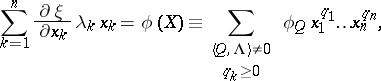where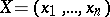andis analytic at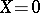(where) and is expressed as the given Taylor series, is given by the Taylor series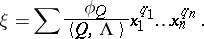This series converges in a neighbourhood of zero if there are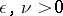such that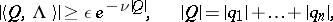(2)

for all integer-valued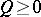,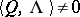. This condition is optimal in the class of all analytic functions; it is necessary for the convergence of the series.

b) Fourier series. The solutionof the equation(3)

whereand the right-hand side is expressed as a Fourier series, is given by the Fourier series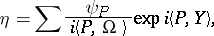which converges in a strip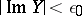ifis analytic and if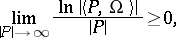(4)

where the limit is taken over all integer-valued,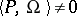. This condition is optimal in the class of all analytic functionsof the form (3).

Equation (3) arises in the reduction of a system of ordinary differential equations on a torus (see ; there (2) is erroneously given instead of (4)). The situation is similar when integrating with respect toa conditionally-periodic function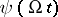. Similar linear problems occur at each approximation in the iterated solution of non-linear problems (in perturbation theory).

If (2) or (4) are not satisfied, then the non-formal solution of the corresponding problem need not be analytic, smooth or need not exist at all (depending on the arithmetic properties of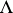and), although formal solutions, the seriesand, always exist (see ).

## 2. Non-linear problems.

In these problems small divisors (1) do not appear singly but in products.

a) Taylor series. Consider a system near a fixed point,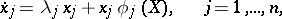(5)

whereis a convergent Taylor series without free term. Letfor integer-valued,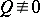. Then there is a formally invertible change of coordinates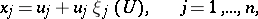where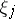is also a Taylor series without free term, which transforms (5) to the normal form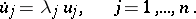(5prm)

The seriesconverges in a neighbourhood of zero if(6)

where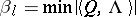for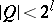,,(see ).

Non-linear problems of this type were first solved by C.L. Siegel (1942; see , ) under the stricter condition: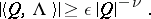(7)

Under this condition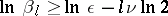and (6) converges. Condition (2) is equivalent to boundedness of the terms of (6); it is necessary for the convergence offor arbitrary analytic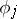. (In  necessity of condition (6) foris claimed; for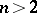it is unknown what happens in the "gap" between the conditions (2) and (6) (for more complicated resonance situations, see ).) If (2) is not satisfied, then between the solutions of (5) and its normal form (5prm) there need not be an analytic, a smooth or even a topological correspondence.

b) Poisson series. Let an analytic system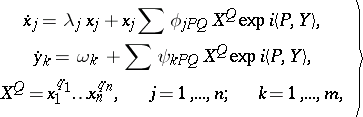(8)

the right-hand sides of which are expanded as a Poisson series near the invariant torus(that is, a Taylor series with respect toand a Fourier series with respect to), have a formal integral manifold(9)

where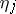is also a Poisson series. The question arises as to when this manifold is analytic (that is, when isabsolutely convergent for sufficiently small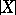and). Here, among the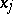there may be small parameters; for them. Such problems were first solved by A.N. Kolmogorov  for the Hamiltonian system (8) withdegrees of freedom and one small parameter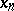(that is,and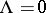): Under the condition(10)

the analyticity of the manifold (9), consisting of invariant tori, was proved for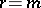. At the same place it was suggested for the first time that "Newton's method" , which is fundamental in research into non-linear problems, be used for the proof of the convergence of the Poisson series. Condition (10) and its analogue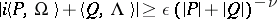were then used in problems of the same type (see ). The conditions (2) and (4) are also necessary here for the convergence of (9) (for more complicated degenerate situations, see ). If these conditions are not satisfied, there need not be an analytic (or even continuous) invariant manifold of the form (9).

The most strict of the restrictions (2), (6), (7), condition (7), is, for, satisfied for almost-all (relative to Lebesgue measure) vectors. Properties of the type of (2), (6), (7) for vectorsare studied in the theory of Diophantine approximations. The two-dimensional case has been rather well studied. Let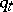be the denominator of the-th convergent of the continued fraction of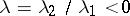. Then (6) is equivalent to convergence of the series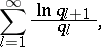and (2) is equivalent to boundedness of its terms (see also , ).

Small divisors (1) with variableandhave been discussed (see ).

Small divisors were first encountered in celestial mechanics, and the fundamental linear problems were solved in 1884 by H. Bruns. In general, in the solar system there are many "points of commensurability" between frequencies, a consequence of which are the small divisors (1). For example, the small divisor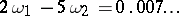, where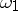andare the frequencies of the motions of Jupiter and Saturn, respectively, leads to the appearance of large reciprocal perturbations in the motions of these planets. Another example: the gaps in the asteroid belt and in Saturn's rings correspond to resonance with the frequencies of the perturbing body (Jupiter and Mimas, respectively).

How to Cite This Entry:
Small denominators. Encyclopedia of Mathematics. URL: http://encyclopediaofmath.org/index.php?title=Small_denominators&oldid=14339
This article was adapted from an original article by A.D. Bryuno (originator), which appeared in Encyclopedia of Mathematics - ISBN 1402006098. See original article